Function Repository Resource:

# RoundedCylinder

Represent a cylinder with rounded edges

Contributed by: Jaroslav Kysela
 ResourceFunction["RoundedCylinder"][{{x1,y1,z1},{x2,y2,z2}},r] represents a rounded cylinder of radius r around the line from (x1,y1,z1) to (x2,y2,z2). ResourceFunction["RoundedCylinder"][{{x1,y1,z1},{x2,y2,z2}}] represents a rounded cylinder of radius 1.

## Details and Options

ResourceFunction["RoundedCylinder"][] is equivalent to ResourceFunction["RoundedCylinder"][{{0,0,-1},{0,0,1}},1].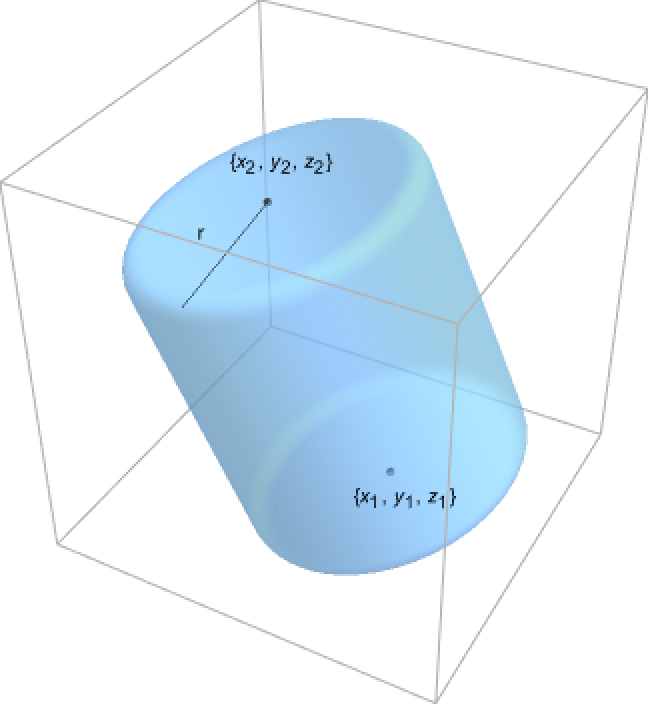ResourceFunction["RoundedCylinder"] can be used in Graphics3D.
Graphics rendering is affected by directives such as FaceForm, Specularity, Opacity, and color.
The following option can be given:

## Examples

### Basic Examples (3)

A unit radius and two units height rounded cylinder:

 In:=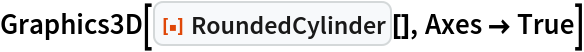Out=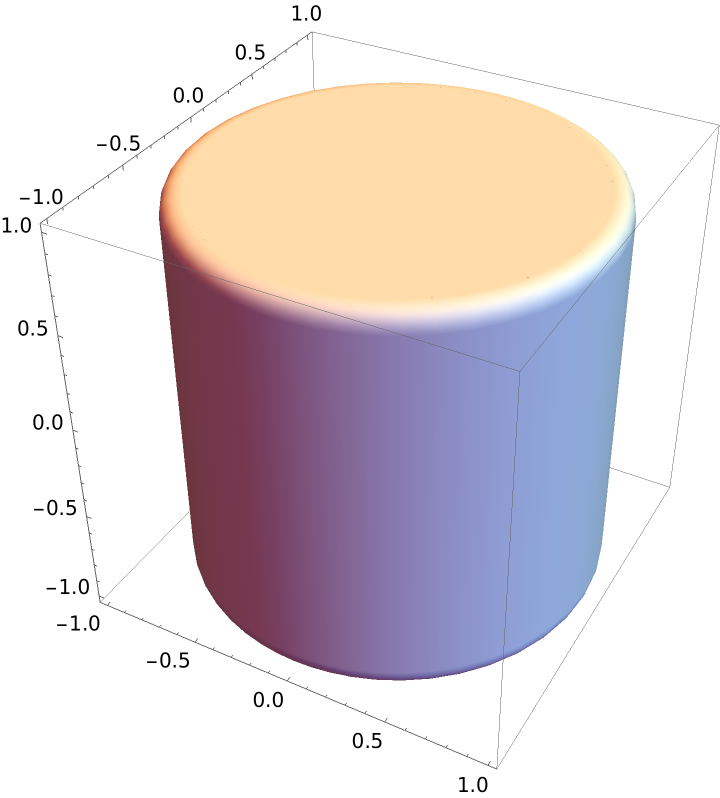A rounded cylinder from the origin to {1,1,1} with radius 1/2:

 In:=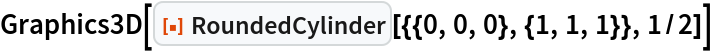Out=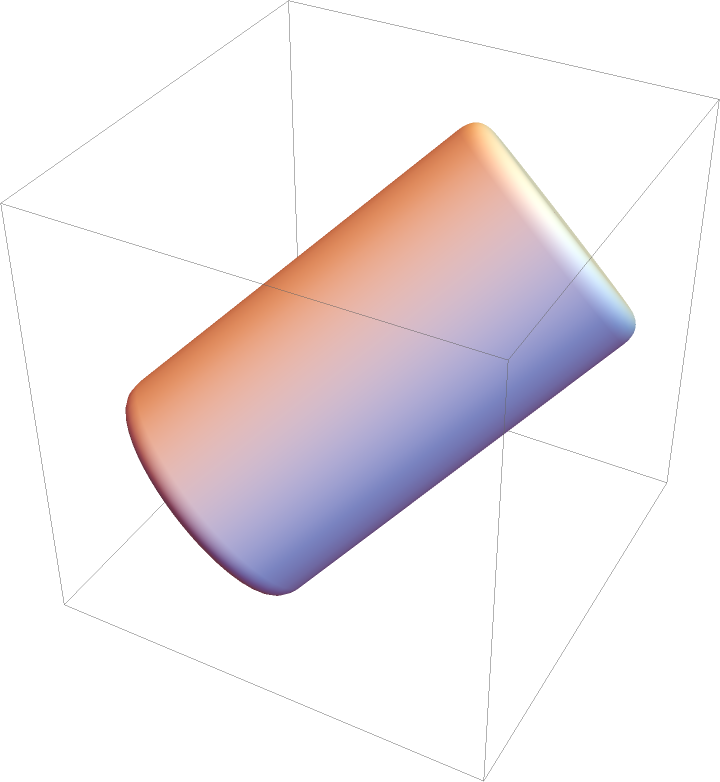Differently styled rounded cylinders:

 In:=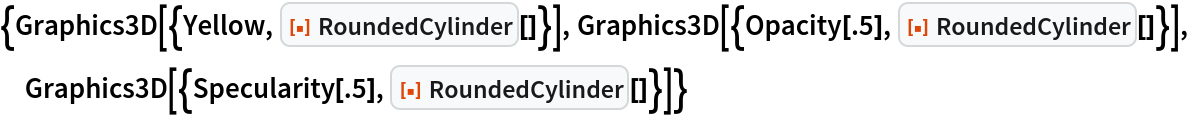Out=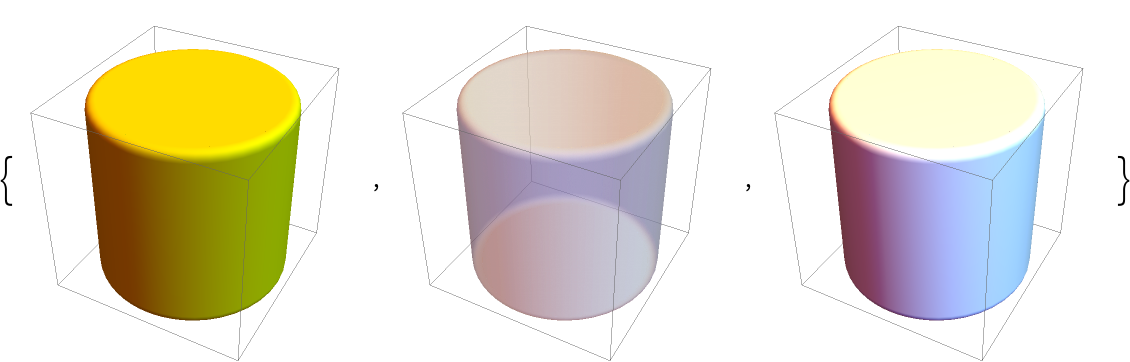### Scope (6)

Rounded cylinders with different endpoints:

 In:=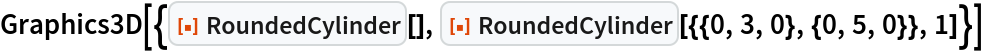Out=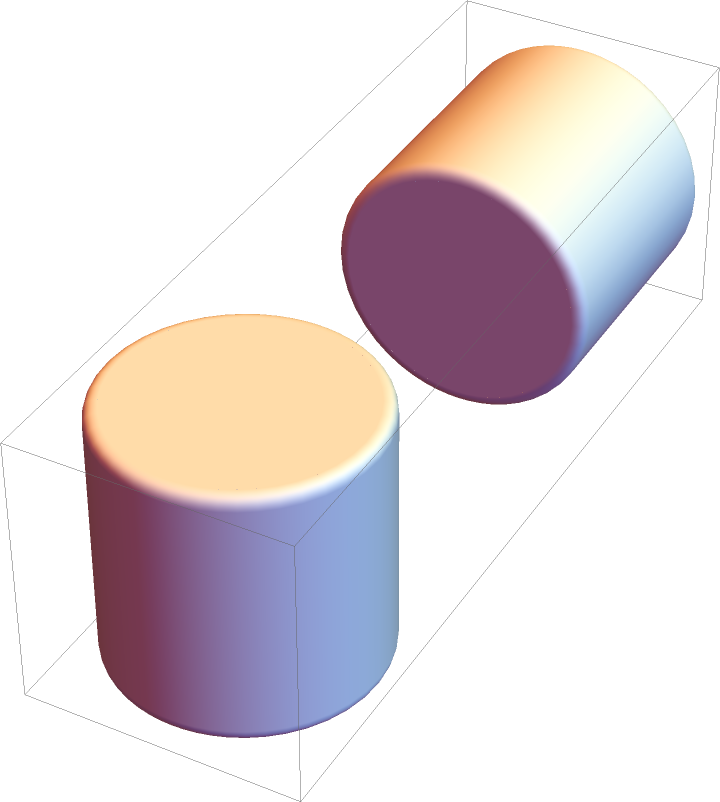In:=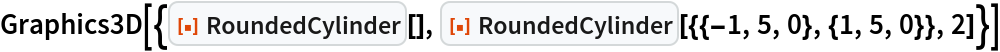Out=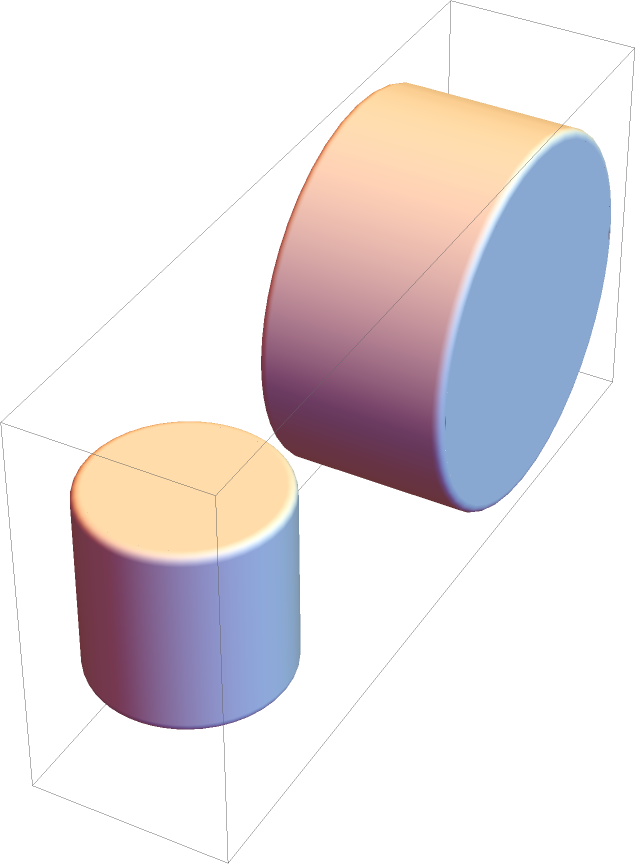Rounded cylinders with different rounding radii:

 In:=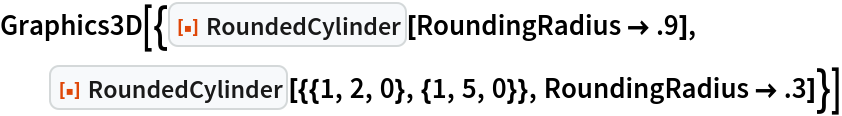Out=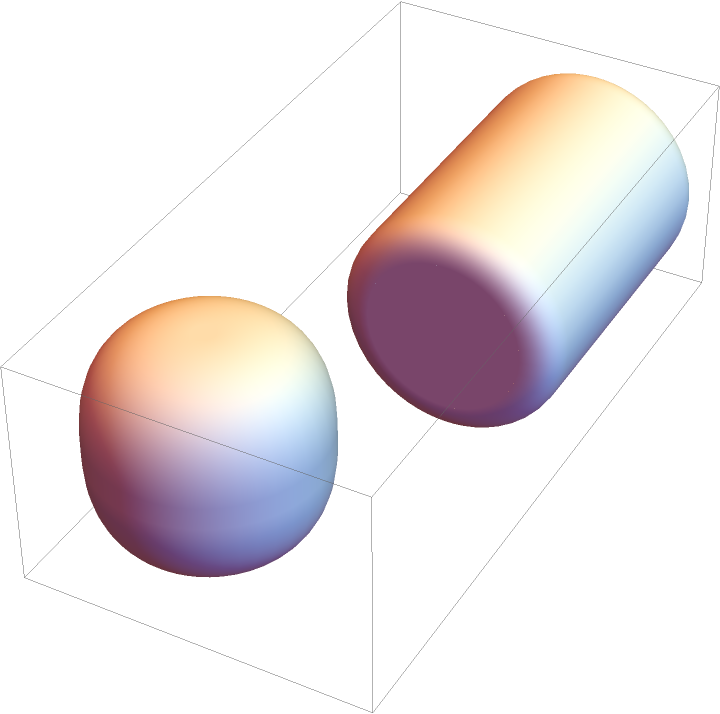Colored cylinders:

 In:=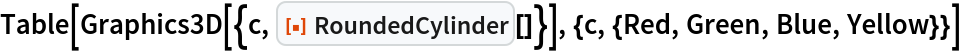Out=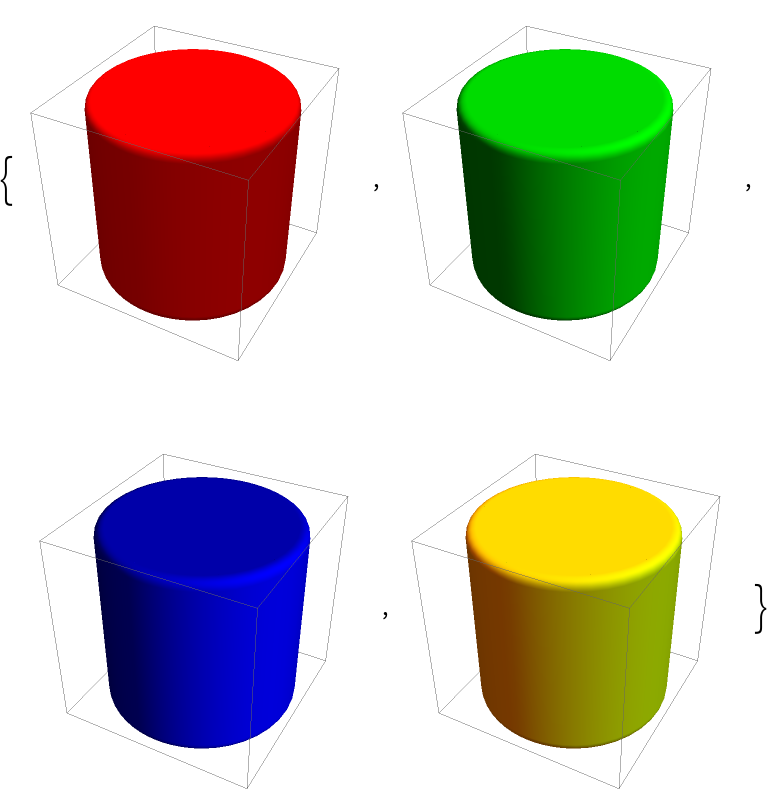Different properties can be specified for the front and back of faces using FaceForm:

 In:=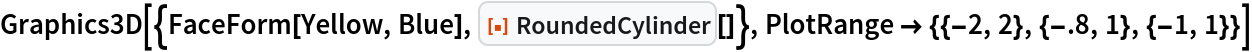Out=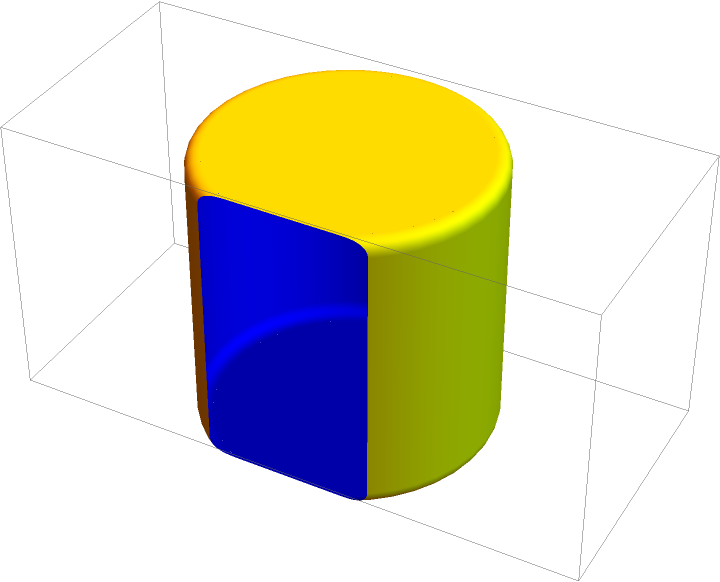Opacity specifies the face opacity:

 In:=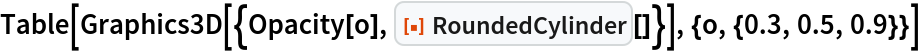Out=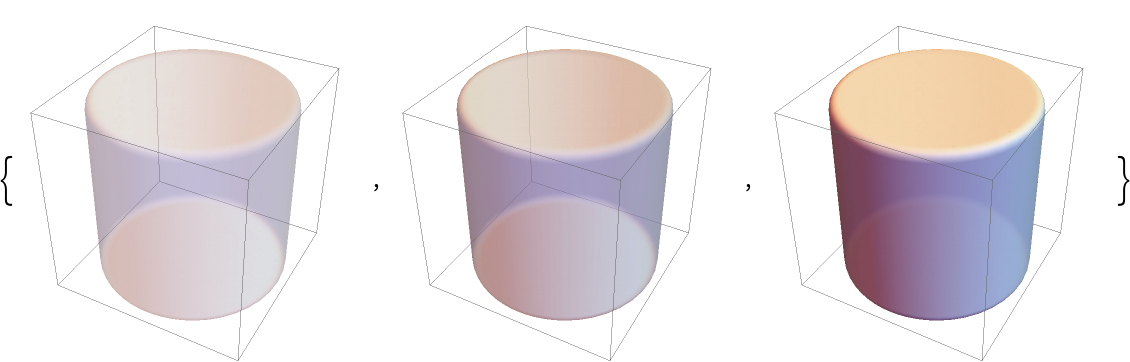### Options (1)

Adjust how rounded the edges are:

 In:=Out=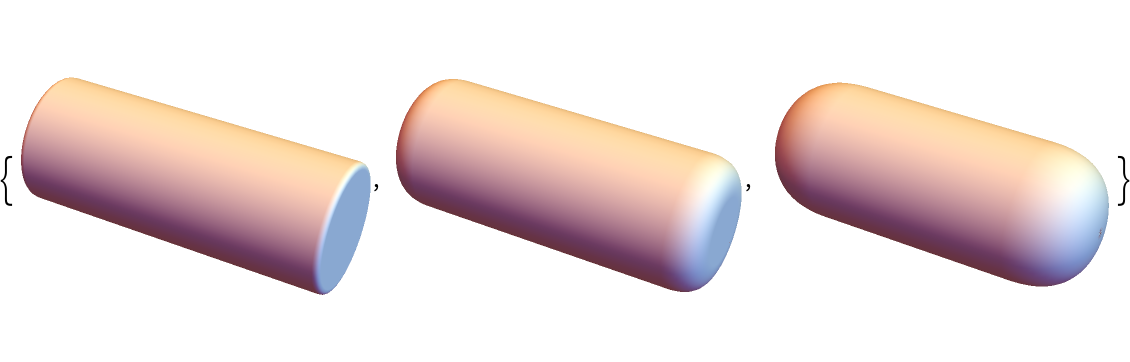### Applications (1)

A simple 3D bar chart:

 In:=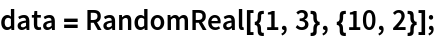In:=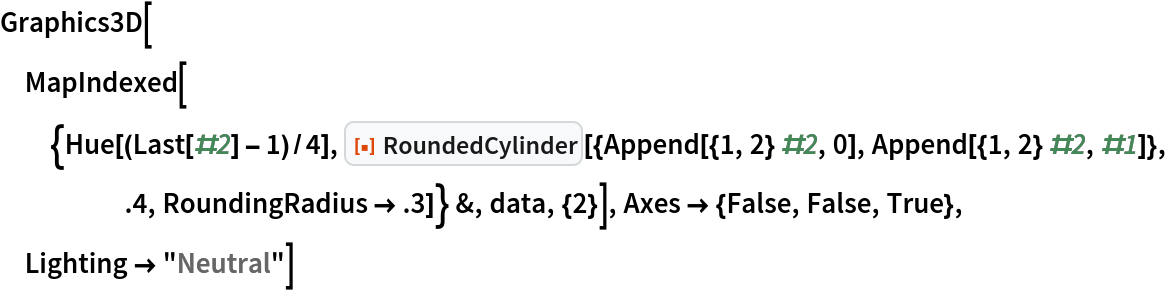Out=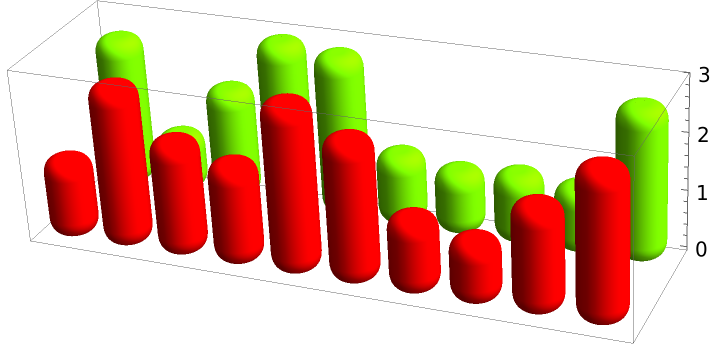### Properties and Relations (2)

For small rounding radii, RoundedCylinder is effectively equivalent to Cylinder:

 In:=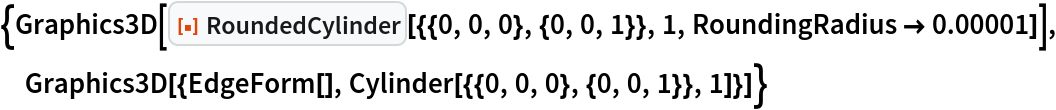Out=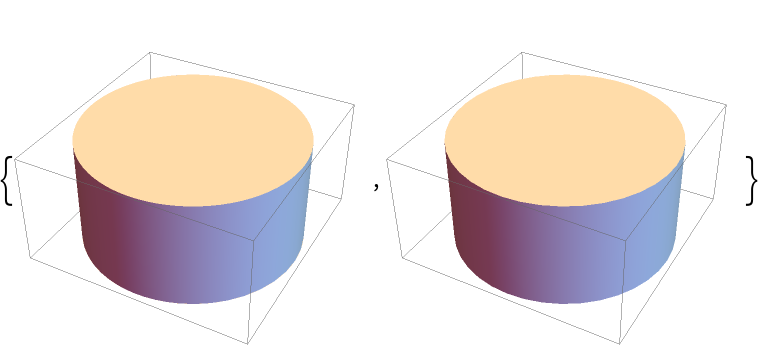When the rounding radius is set to the cylinder radius, RoundedCylinder closely resembles CapsuleShape as well as Tube with rounded caps:

 In:=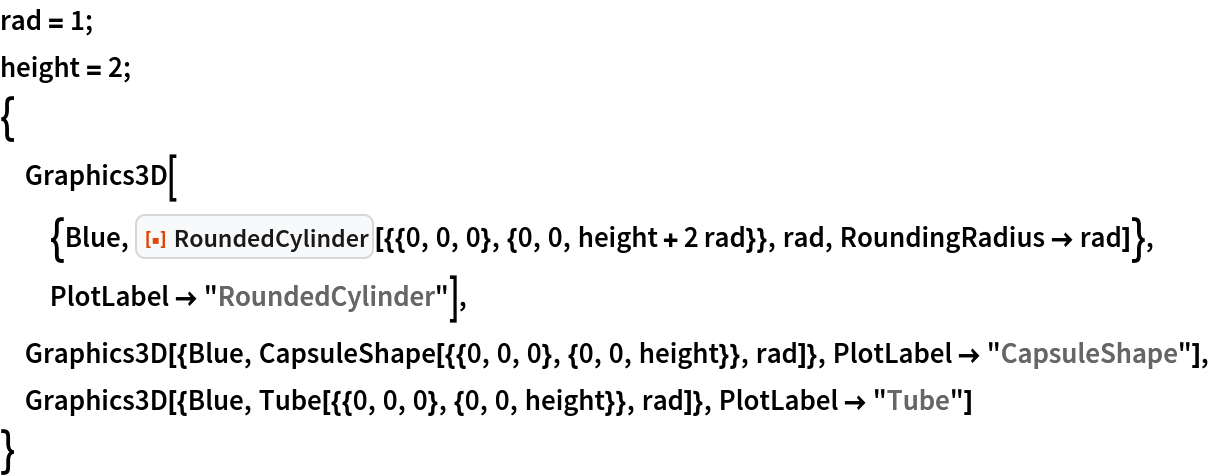Out=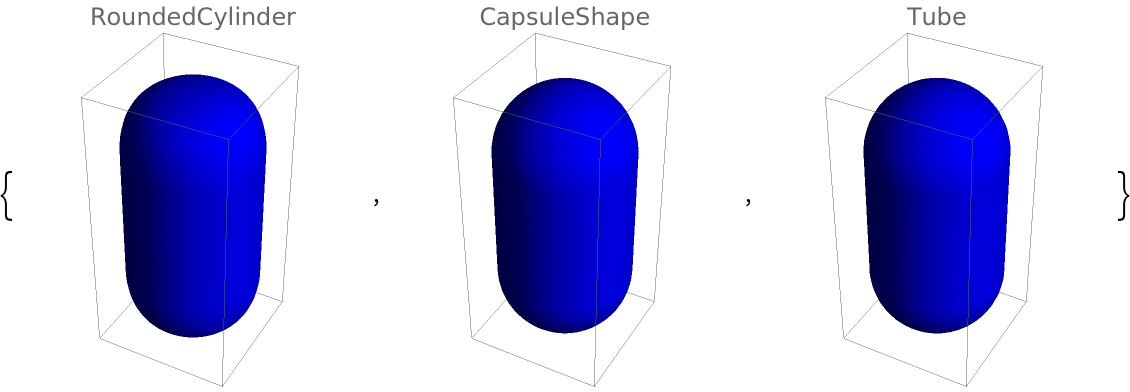### Possible Issues (3)

When the two endpoints coincide, no cylinder is created:

 In:=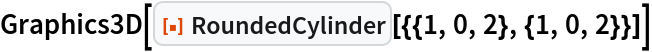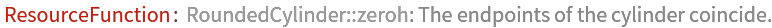Out=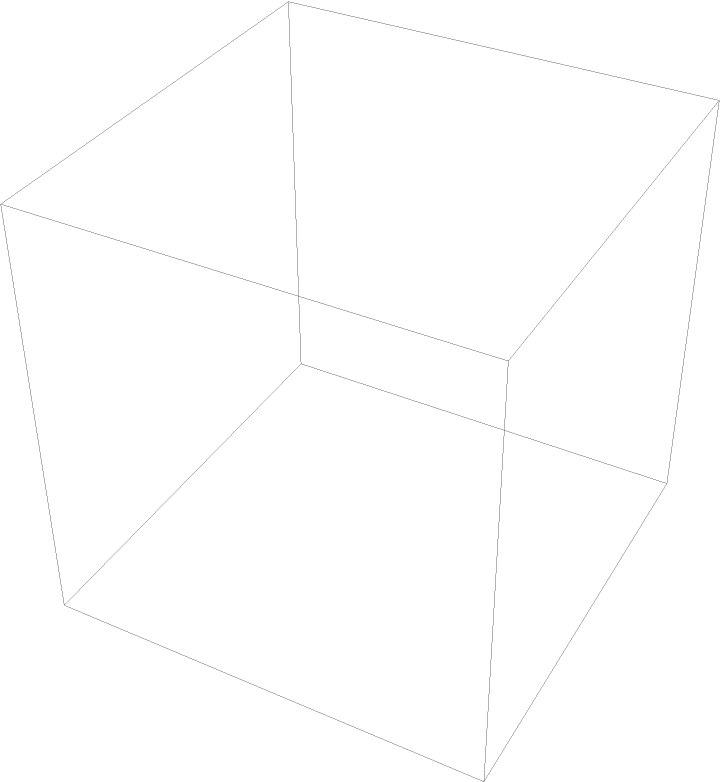When the rounding radius exceeds that of the cylinder, a warning is issued and artifacts appear:

 In:=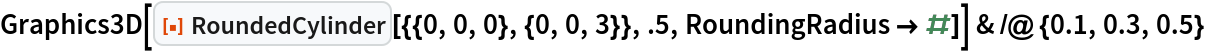Out=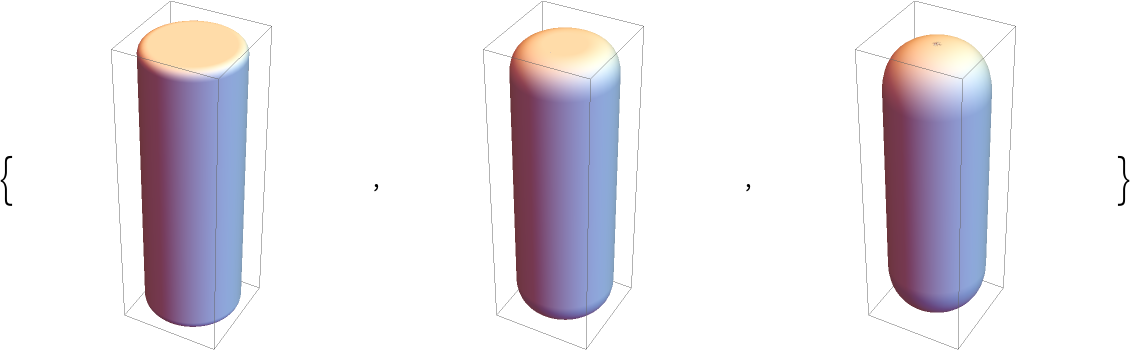In:=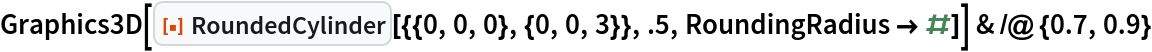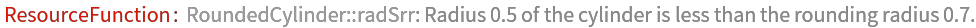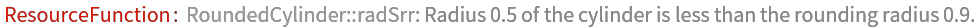Out=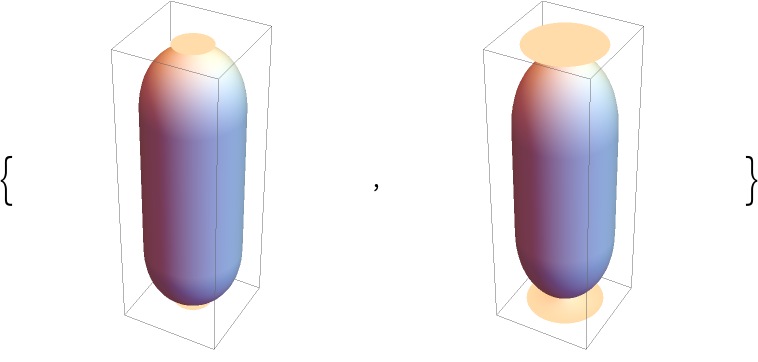When the double of the rounding radius exceeds the height of the cylinder, a warning is issued and artifacts appear:

 In:=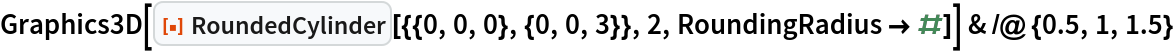Out=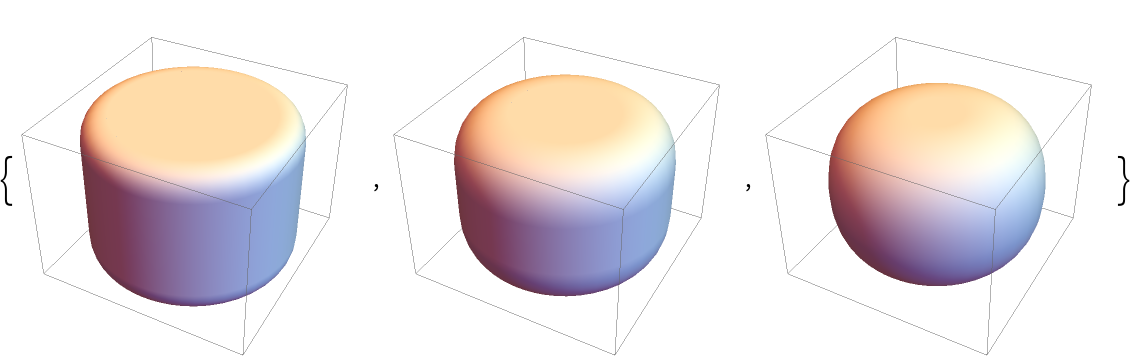In:=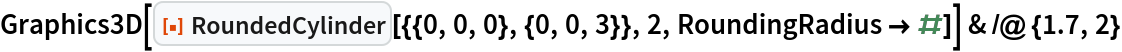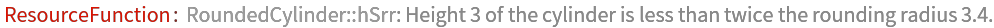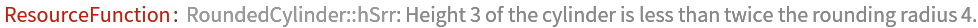Out=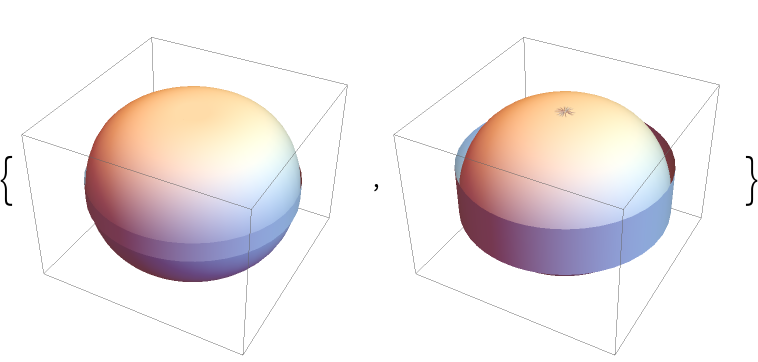### Neat Examples (2)

Random unit rounded cylinders:

 In:=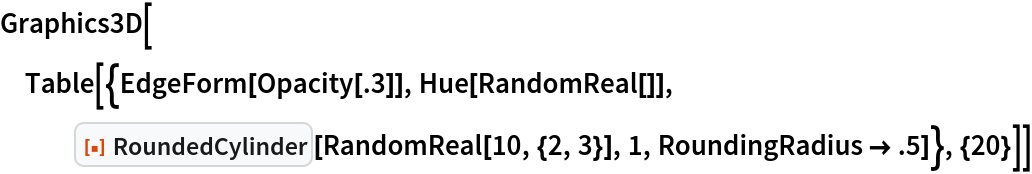Out=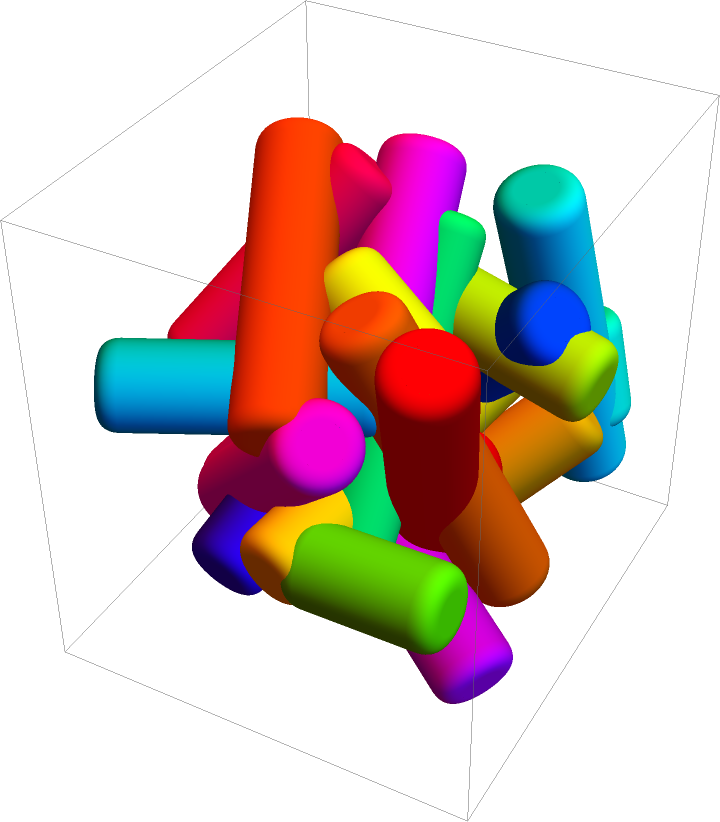Bouncing cylinders:

 In:=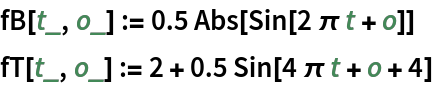In:=In:=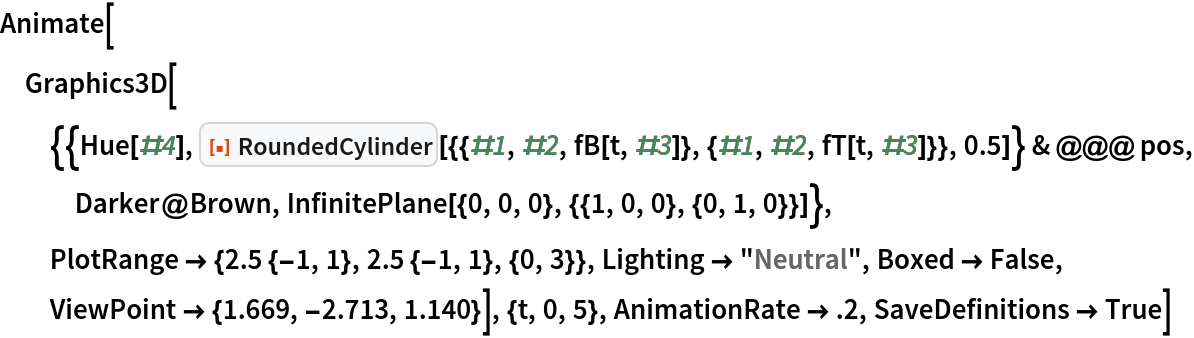Out=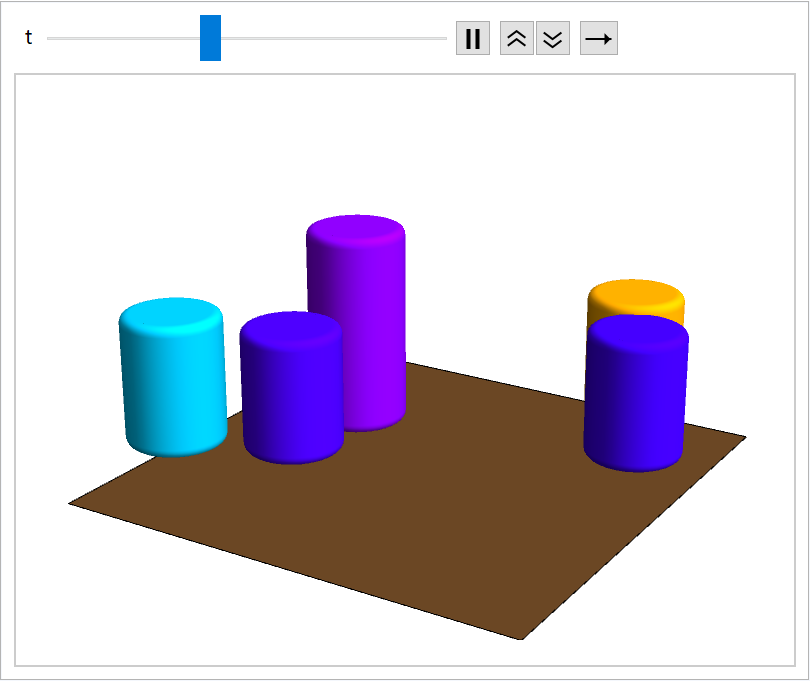Jaroslav Kysela

## Version History

• 1.0.0 – 20 December 2021